# Borel summation method

A method for summing series of functions, proposed by E. Borel . Suppose one is given a series of numbers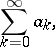(*)

let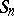be its partial sums and letbe a real number. The series (*) is summable by the Borel method (-method) to the numberif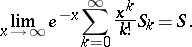There exists an integral summation method due to Borel. This is the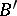-method: If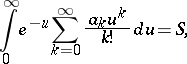then one says that the series (*) is summable by the-method to the number. For conditions under which the two methodsandare equivalent, cf. . The-method originated in the context of analytic extension of a function regular at a point. Let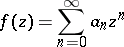be regular at the point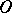and let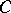be the set of all its singular points. Draw the segmentand the straight line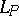normal to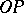through any point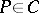. The set of points on the same side withfor each straight lineis denoted by; the boundaryof the domainis then called the Borel polygon of the function, while the domain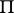is called its interior domain. The following theorem is valid: The seriesis summable by the-method in, but not in the domainwhich is the complement of.